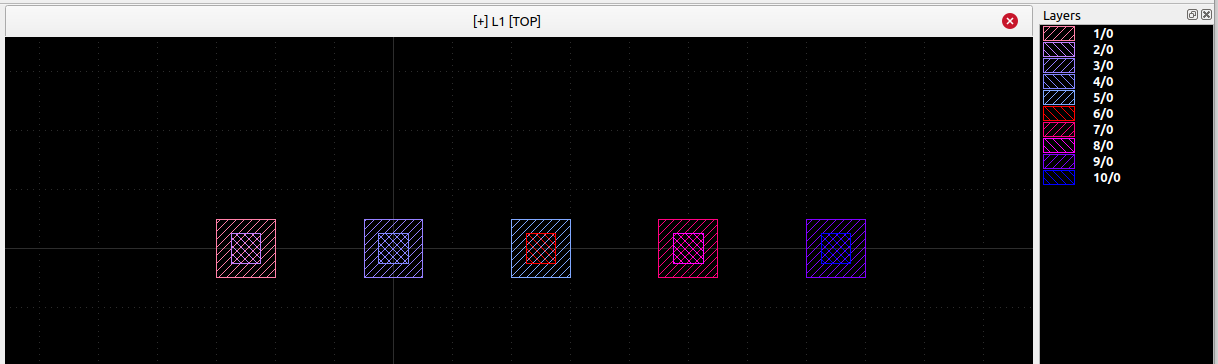# Creating arrays of layers from a design of multiple layers

Hi. I would like to do something like. Draw N boxes on layers 1,2,3.....,N in the same drawing. Then this would be a ??cell?? ??Pcell??... I then would like to make a new drawing where it has M layers 1,2,3.....,M of the ??cell?? ??PCell??.
As a definitive example. Imagine I wanted to draw 2 overlapping boxes on layers 1 and 2 (N=2), layer 1 has a box 10umx10um, layer 2 has a box centred at centre of layer 1 but is only 5umx5um. I then somehow save this drawing and draw it 5 times with an offset of 25um such that there are now 5 layers (perhaps 10 if significantly easier) in my drawing and every box (big and small) is of a different layer such that I can differentiate between them on an external piece of software.

• Are you looking for something like this?This is the Python code which generates that:

``````# puts 5 boxes on 10 different layers in the current view

ly = pya.CellView.active().layout()

for l in range(0, 5):

x = (l - 1) * 25.0
y = 0

# 10x10 um box at x,y on layer l1 (1/0, 3/0, ...)
l1 = ly.layer(l * 2 + 1, 0)
ly.top_cell().shapes(l1).insert(pya.DBox(-5.0, -5.0, 5.0, 5.0).moved(x, y))

# 5x5 um box at x,y on layer l2 (2/0, 4/0, ...)
l2 = ly.layer(l * 2 + 2, 0)
ly.top_cell().shapes(l2).insert(pya.DBox(-2.5, -2.5, 2.5, 2.5).moved(x, y))